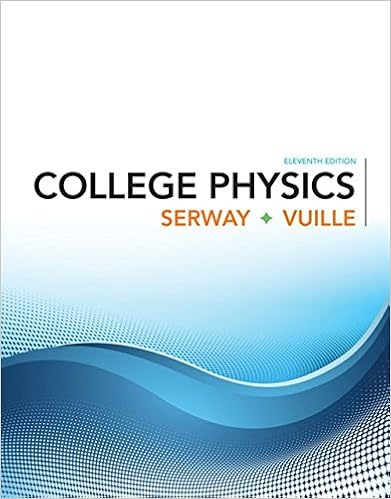# Thus to find all points of intersection of two polar

• Notes
• mimi304
• 54
• 40% (5) 2 out of 5 people found this document helpful

This preview shows page 33 - 36 out of 54 pages.

##### We have textbook solutions for you!
The document you are viewing contains questions related to this textbook.The document you are viewing contains questions related to this textbook.
Chapter 1 / Exercise 1.3
College Physics
Serway/VuilleExpert Verified
Thus, to find all points of intersection of two polar curves, it is recommended that you draw the graphs of both curves. It is especially convenient to use a graphing calculator or computer to help with this task. Find all points of intersection of the curves and . SOLUTION If we solve the equations and , we get and, there- fore, , , , . Thus the values of between and that satisfy both equations are , , , . We have found four points of inter- section: , , and . However, you can see from Figure 7 that the curves have four other points of inter- section—namely, , , , and . These can be found using symmetry or by noticing that another equation of the circle is and then solving the equations and . Arc Length To find the length of a polar curve , , we regard as a parameter and write the parametric equations of the curve as Using the Product Rule and differentiating with respect to , we obtain r f r t a b f t 0 0 b a 2 A r t r f A y b a 1 2 f 2 d y b a 1 2 t 2 d 1 2 y b a ( f 2 t 2 ) d r 3 sin r 1 sin ( 3 2 , 6 ) ( 3 2 , 5 6 ) 0, 0 0, r 3 sin 0, 3 2 r 1 sin 2 0 3 2 r cos 2 r 1 2 r cos 2 r 1 2 cos 2 1 2 2 3 5 3 7 3 11 3 0 2 6 5 6 7 6 11 6 ( 1 2 , 6 ) ( 1 2 , 5 6 ) , ( 1 2 , 7 6 ) ( 1 2 , 11 6 ) ( 1 2 , 3 ) ( 1 2 , 2 3 ) ( 1 2 , 4 3 ) ( 1 2 , 5 3 ) r 1 2 r cos 2 r 1 2 EXAMPLE 3 r f a b x r cos f cos y r sin f sin dx d dr d cos r sin dy d dr d sin r cos O ¨=b ¨=a r=f(¨) r=g(¨) FIGURE 6 FIGURE 7 r= cos  2¨ 1 2 r= ”   ,     ’ 1 2 π 3 ”   ,    ’ 1 2 π 6
##### We have textbook solutions for you!
The document you are viewing contains questions related to this textbook.The document you are viewing contains questions related to this textbook.
Chapter 1 / Exercise 1.3
College Physics
Serway/VuilleExpert Verified
692 CHAPTER 10 so, using , we have Assuming that is continuous, we can use Theorem 10.2.5 to write the arc length as Therefore the length of a curve with polar equation , , is Find the length of the cardioid . SOLUTION The cardioid is shown in Figure 8. (We sketched it in Example 7 in Section 10.3.) Its full length is given by the parameter interval , so Formula 5 gives We could evaluate this integral by multiplying and dividing the integrand by , or we could use a computer algebra system. In any event, we find that the length of the cardioid is . dr d 2 sin 2 2 r dr d sin cos r 2 cos 2 dr d 2 r 2 f L y b a dx d 2 dy d 2 d cos 2 sin 2 1 dx d 2 dy d 2 dr d 2 cos 2 2 r dr d cos sin r 2 sin 2 r f a b 5 L y b a r 2 dr d 2 d r 1 sin 0 2 L y 2 0 r 2 dr d 2 d y 2 0 s 1 sin 2 cos 2 d y 2 0 s 2 2 sin d s 2 2 sin L 8 v EXAMPLE 4 O FIGURE 8 r=1+ sin  ¨ ; Graphing calculator or computer required 1. Homework Hints available at stewartcalculus.com 1–4 Find the area of the region that is bounded by the given curve and lies in the specified sector. 1. , 2. , 3. , , 4. , r e 4 2 r cos 0 6 r 2 9 sin 2 0 2 r tan 6 3 r 0 5–8 Find the area of the shaded region. 5. 6. r=œ ¨ r=1+ cos  ¨ 10.4 Exercises
AREAS AND LENGTHS IN POLAR COORDINATES 693 7. 8. 9–12 Sketch the curve and find the area that it encloses. 9. 10. 11. 12. ; 13–16 Graph the curve and find the area that it encloses. 13. 14. 15. 16. 17–21 Find the area of the region enclosed by one loop of the curve.
•••